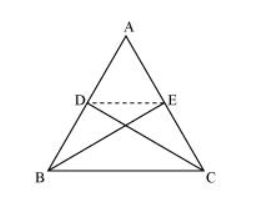# D and E are points on sides AB and AC respectively of ΔABC such thatQuestion. D and E are points on sides AB and AC respectively of ΔABC such that ar (DBC) = ar (EBC). Prove that DE || BC.

Solution:Since ΔBCE and ΔBCD are lying on a common base BC and also have equal areas, ΔBCE and ΔBCD will lie between the same parallel lines.

$\therefore \mathrm{DE} \| \mathrm{BC}$